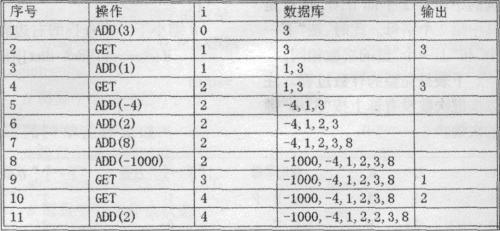## P1801 黑匣子[堆]

### 题目描述

Black Box是一种原始的数据库。它可以储存一个整数数组，还有一个特别的变量i。最开始的时候Black Box是空的．而i等于0。这个Black Box要处理一串命令。

GET:i加1，然后输出Blackhox中第i小的数。1.A(1)，A(2)，…A(M)：一串将要被放进Black Box的元素。每个数都是绝对值不超过2000000000的整数，M\$200000。例如上面的例子就是A=(3，1，一4，2，8，-1000，2)。

2.u(1)，u(2)，…u(N)：表示第u(j)个元素被放进了Black Box里后就出现一个GET命令。例如上面的例子中u=(l，2，6，6)。输入数据不用判错。

### 参考代码

``````#include<cstdio>
#include<iostream>
#include<cmath>
#include<cstring>
#include<ctime>
#include<cstdlib>
#include<algorithm>
#include<queue>
#include<set>
#include<map>
#define ll long long
#define N 200010
using namespace std;
{
int f=1,x=0;char c=getchar();
while(c<'0'||c>'9'){if(c=='-')f=-1;c=getchar();}
while(c>='0'&&c<='9'){x=x*10+c-'0';c=getchar();}
return x*f;
}
priority_queue<ll> Q;
priority_queue<ll,vector<ll>,greater<ll> > q;
int k,a[N],b[N],n,m;
int main()
{
k=1;
Q.push(a);
for(int i=1;i<=m;++i){
if(i>1){
if(a[i]>Q.top()) q.push(a[i]);
else Q.push(a[i]);
}
while(i==b[k]){
while(Q.size()>k) q.push(Q.top()),Q.pop();
while(Q.size()<k) Q.push(q.top()),q.pop();
++k,printf("%d\n",Q.top());
}
}
return 0;
}``````